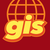# Using Arcade to count nulls or non-nulls from an array

266
2
Jump to solution
02-20-2020 09:17 AMNew Contributor II

Hi,

I am trying to transfer some code I use in python to arcade in order to take advantage of the attribute rule calculation. I currently have some python that calculates the average of an array but discounts null values if it finds them in the array. I'm looking to use arcade to count how many nulls or non-nulls there are... the Count function or the isEmpty function don't seem suited.

The last best code which isn't working (so best isn't great!) is:

var array = [\$feature.Welcoming,\$feature.Access];
var count = [i for i in array if i];
'Sum(array) / float(count)'

Any help would be great thanks!

1 Solution

Accepted SolutionsbyEsri Esteemed Contributor

Hi Jim Phillips ,

Have a look at the snippet below:

``var arr = [1,null,3];Console("sum :" + Sum(arr));Console("mean:" + Mean(arr));var tot = 0;var cnt = 0;for (var i in arr) {    if (!IsEmpty(arr[i])) {        tot+=arr[i];        cnt+=1;    }}var promedio = tot/cnt;return promedio;‍‍‍‍‍‍‍‍‍‍‍‍‍‍‍``

I have an array with 3 values, of which 1 is null. When I use the Sum it will return a correct result, since 1+null+3 = 4. The Mean however will not return the correct result, since the sum 4 will be divided by 3 elements and return 1.333.

To avoid this, you will probably have to loop through the array and only cnt and sum the non-null values. In Arcade you cannot use the list comprehensions that you are showed in your example.

2 RepliesbyEsri Esteemed Contributor

Hi Jim Phillips ,

Have a look at the snippet below:

``var arr = [1,null,3];Console("sum :" + Sum(arr));Console("mean:" + Mean(arr));var tot = 0;var cnt = 0;for (var i in arr) {    if (!IsEmpty(arr[i])) {        tot+=arr[i];        cnt+=1;    }}var promedio = tot/cnt;return promedio;‍‍‍‍‍‍‍‍‍‍‍‍‍‍‍``

I have an array with 3 values, of which 1 is null. When I use the Sum it will return a correct result, since 1+null+3 = 4. The Mean however will not return the correct result, since the sum 4 will be divided by 3 elements and return 1.333.

To avoid this, you will probably have to loop through the array and only cnt and sum the non-null values. In Arcade you cannot use the list comprehensions that you are showed in your example.New Contributor II

Brilliant, I'm starting to understand Arcade a bit. Your snippet works perfectly, really helpful.